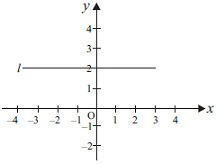# User Forum

Subject :IMO    Class : Class 9

Study the given graph and answer the following question.Calculate the area enclosed by the lines l, x = -3, y = -2 and y = -x + 2.

A16 sq. units
B19 sq. units
C20 sq. units
D22 sq. units

If any of you have the answer for this, please write it over here